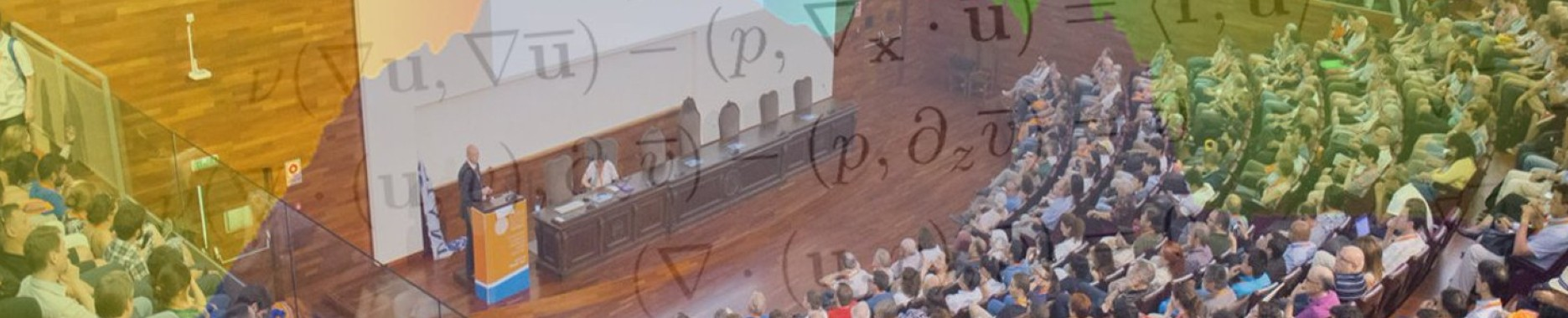## Salón de Actos

Speaker: José M. Mazón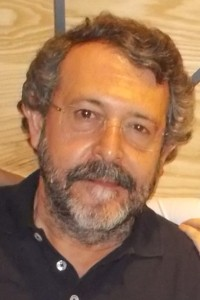Title: Gradient flows in metric random walk spaces

Abstract: In our digital world many different kinds of data are now available (images, social networks, etc). To represent these data the most natural and flexible representation consist in using weighted graphs. Historically the main tools for the study of graphs came from combinatorial graph theory. Recently, there has been increasing interest in the research of partial differential equations (PDE’s) in graphs. On the other hand, in recent years there has been a great development of the study of nonlocal PDE’s motivated by problems in image processing, the analysis of the peridynamic formulation of the continuous mechanic, Markov jump processes, etc. In the last years we have studied some gradient flows in the general framework of metric random walk spaces, that is, metric spaces together with a probability measure assigned to each point of the space that encode the jumps of a Markov process, that include, in particular, PDE’s in weighted graphs and some nonlocal PDE’s. In this lecture we summarize these results.

Speaker: David Ruiz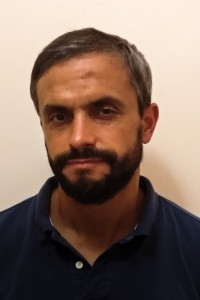Title: Traveling waves for the Gross-Pitaevskii equation in the subsonic regime

Abstract: The Gross-Pitaevskii equation is a Schrödinger equation under the effect of a Ginzburg-Landau potential, and has been proposed to study different phenomena like Bose-Einstein condensation. In this talk we will first study the qualitative behavior of traveling waves, like uniform boundedness, regularity and asymptotic behavior.

Regarding existence, some previous existence results have been obtained via a constrained minimization procedure. This has the advantage of providing orbital stability of the solutions but, as a drawback, the speed of propagation appears as a Lagrange multiplier and is not controled. We will present a recent general existence result for almost all speeds in the subsonic regime.

Speaker: Tere M-SearaTitle: Breakdown of small amplitude breathers for some Klein-Gordon equations

Abstract: Breathers are periodic in time spatially localized solutions of evolutionary PDEs. They are known to exist for the sine-Gordon equation but are believed to be rare in other Klein-Gordon equations. Breathers can be seen as homoclinic solutions to a steady solution in an infinite dimensional space. In this talk, we prove an asymptotic formula for the distance between the stable and unstable manifold of the steady solution when the steady solution has weakly hyperbolic one dimensional stable and unstable manifolds. This formula allows to say that for a wide set of Klein-Gordon equations breathers do not exist. The distance is exponentially small with respect to the amplitude of the breather and therefore classical perturbative techniques, like Melnikov theory, cannot be applied.

This is a joint work with O. Gomide, M: Guardia and C. Zeng.

Speaker: Daniel Peralta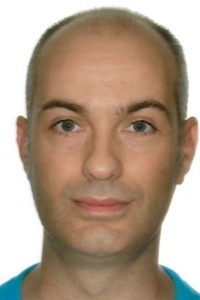Title: Vortex reconnections in classical and quantum fluids.

Abstract:

The evolution of a classical viscous fluid is described by the Navier-Stokes equations, while the dynamics of a quantum fluid (or Bose-Einstein condensate) is modelled by the Gross-Pitaevskii equation. In both cases the dynamics of the vortex lines plays a fundamental role in the analysis of the equations.

Recent experiments and extensive numerical computations show that the vortices of both classical and quantum fluids cross during their evolution, each of them breaking into two parts and reconnecting part of itself for part of the other. This phenomenon, known as vortex reconnection, occurs even though the fluid does not lose its smoothness.

In this talk, I will show how classical and quantum vortices, of arbitrarily complicated topologies, are created and destroyed in smooth solutions to the corresponding equations. Key to prove this result is a new inverse localization principle for Beltrami fields and a novel flexibility property of the linear Schrodinger equation.

This talk is based on joint works with Alberto Enciso, Renato Lucà and Francisco Torres de Lizaur.

Speaker: Boris Vexler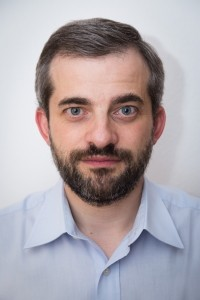Title: Numerical Analysis of sparse initial data identification for parabolic problems

Abstract: We consider a problem of identification of initial data for a homogeneous parabolic equation from an observation of the final state.

In general, such problems are known to be exponentially ill-posed.  We are interested in the situation, where the initial data, we are looking for, is known to be sparse, i.e. to have a support of Lebesgue measure zero. The strong smoothing property of parabolic equations makes it difficult to identify such sparse initial data. The remedy is the incorporation of the information that the unknown initial data should be sparse into the optimal control formulation. This allows for robust identification of initial data consisting of finitely many Dirac impulses with unknown number and positions. For the resulting optimal control problem we discuss finite element discretization and provide precise error analysis.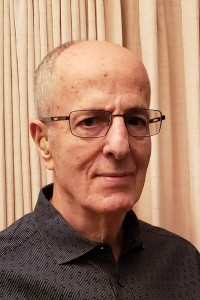Title:  Numerical Linear Algebra for data-related applications

Abstract: Numerical linear algebra is undergoing momentous changes as it starts to cope with the problems that arise from applications related to machine learning and, generally, from data-centered methodologies.  A common tool that is exploited in many methods that rely on large data sets is that of dimension reduction'.  Dimension reduction is based on the precept that the observed data often lies in a noisy version of a low-dimensional subspace and so it is critical to work in this subspace not only to reduce computational cost but also to improve accuracy.  The talk will start with an overview of the key concepts and then illustrate dimension reduction methods with applications such as information retrieval, face recognition and matrix completion for recommender systems. It will also illustrate the important role that graph Laplaceans, and other graph theory tools, play in applications that range from clustering and image segmentation, to the analysis of networks.

Speaker: Bernardo Cockburn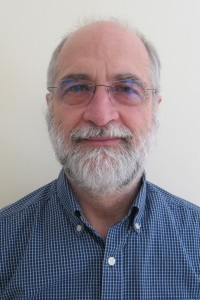Title: Variational principles for HDG methods

Abstract:

We propose a framework, based on variational principles, for the devising of general Galerkin methods for a second-order elliptic model equation. The framework is based on the classic energy and on the complementary energy minimization principles. For each of these priciples, three additional formulations are obtained by relaxing continuity constraints and by relaxing constitutive relations. This framework, which displays eight variational formulations, allows us to place and compare the development of the HDG methods with that of many other methods including the continuous Galerkin, the mixed and the old hybrid methods. Special attention will be put on the contributions that Francisco-Javier Sayas made to the development of the HDG methods. I will also share photos and stories which, I hope, will bring us closer to who was Francisco-Javier, a man of grit, of service, of passion, great to play with, and a talented mathematician whose life was tragically, and untimely, cut short.

Speaker: Salim Meddahi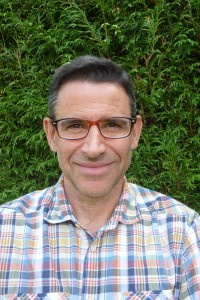Title: Mixed Finite Element Methods in Elastodynamics

Abstract:

The Cauchy stress tensor is a variable of primary interest in many elasticity problems. Great efforts had been made to design stable mixed finite elements for a direct approximation of this field in steady state elasticity problems.

Does these mixed finite elements provide spectrally correct approximations of elasticity eigenproblems? How can the mixed formulation of time harmonic elastodynamic problems be analyzed? What can be done to solve time dependent problems? We will address answers to these questions and extend our discussion to deal with the interaction between a deformable structure and an internal or surrounding acoustic fluid.

Speaker: Marco Ellero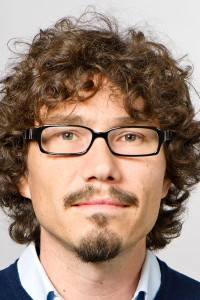Title: GENERIC-compliant stochastic particle models of discrete hydrodynamics

Abstract:

Fluids at the macroscopic scales can be regarded as a continuum and, under certain approximations, evolution equations for fields can be derived in the form of partial differential equations (PDE) reflecting local conservation laws (e.g. mass, momentum, energy). Navier-Stokes equations, for example, represent an accurate model for simple Newtonian fluids and their discretization using classical computational fluid dynamics techniques (e.g. Finite Difference, Finite Elements) represents a formal way to translate those general laws into a discrete setting. Conservation laws are therefore properties embedded into the original PDE, whereas convergence of the numerical scheme towards the exact continuum limit implies the thermodynamic consistency of the computed solution.

In this talk I will discuss a different strategy in which physical laws are enforced "a priori" into discrete particle models of hydrodynamics, and their validity does not formally require a link to the underlying PDE (although it is useful to have it). In particular, I will present the so-called Smoothed Dissipative Particle Dynamics (SDPD) method, a stochastic particle-based technique for fluctuating hydrodynamics.  Application of the GENERIC formalism (General Equation for Non-Equilibrium Reversible Irreversible Coupling) allows to derive a stochastic particle model maintaining its thermodynamic consistency, i.e. enforcement of First/Second Laws of Thermodynamics and Fluctuation-Dissipation Theorem, exactly at the level of the spatial discretization. Beside simple fluids, examples will be given for more general non-Newtonian viscoelastic liquids (e.g. polymer suspensions) as well as for the simulation of complex particulate systems.Title: Communicability function in complex networks. Theory and applications

Abstract: I will start with a gentle introduction to the study of complex networks. Then, I will motivate the problem of "information" transmission in such complex systems. I will provide evidence against the use of shortest topological paths as the main routes for the transmission of information in complex systems. Then, I will introduce the concept of network communicability and its connection with the theory of functions of the adjacency matrix, in particular with the exponential of such matrix. I will show several ways of interpreting the communicability function in complex networks using: (i) combinatorial theory, (ii) networks of coupled harmonic oscillators, (iii) diffusion on networks, (iv) epidemic propagation on networks. I will then show how the communicability function induces an embedding of a given network into a Euclidean hypersphere. Finally, I will illustrate a few applications of the communicability function in the study of several problems in networks, ranging from detection of human brain diseases to the deformation of granular materials.

## Organiza

••## Colabora

•••••